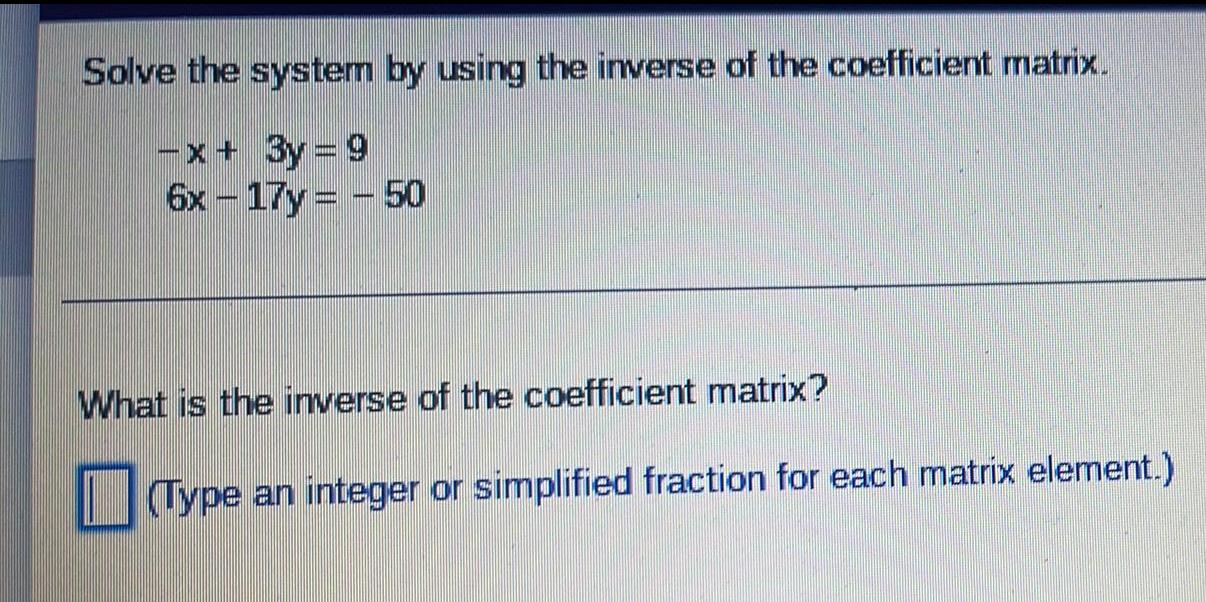Question:

# Solve the system by using the inverse of the coefficient

Last updated: 8/11/2022Solve the system by using the inverse of the coefficient matrix. -x+ 3y=9 6x-17y - 50 What is the inverse of the coefficient matrix? (Type an integer or simplified fraction for each matrix element.)